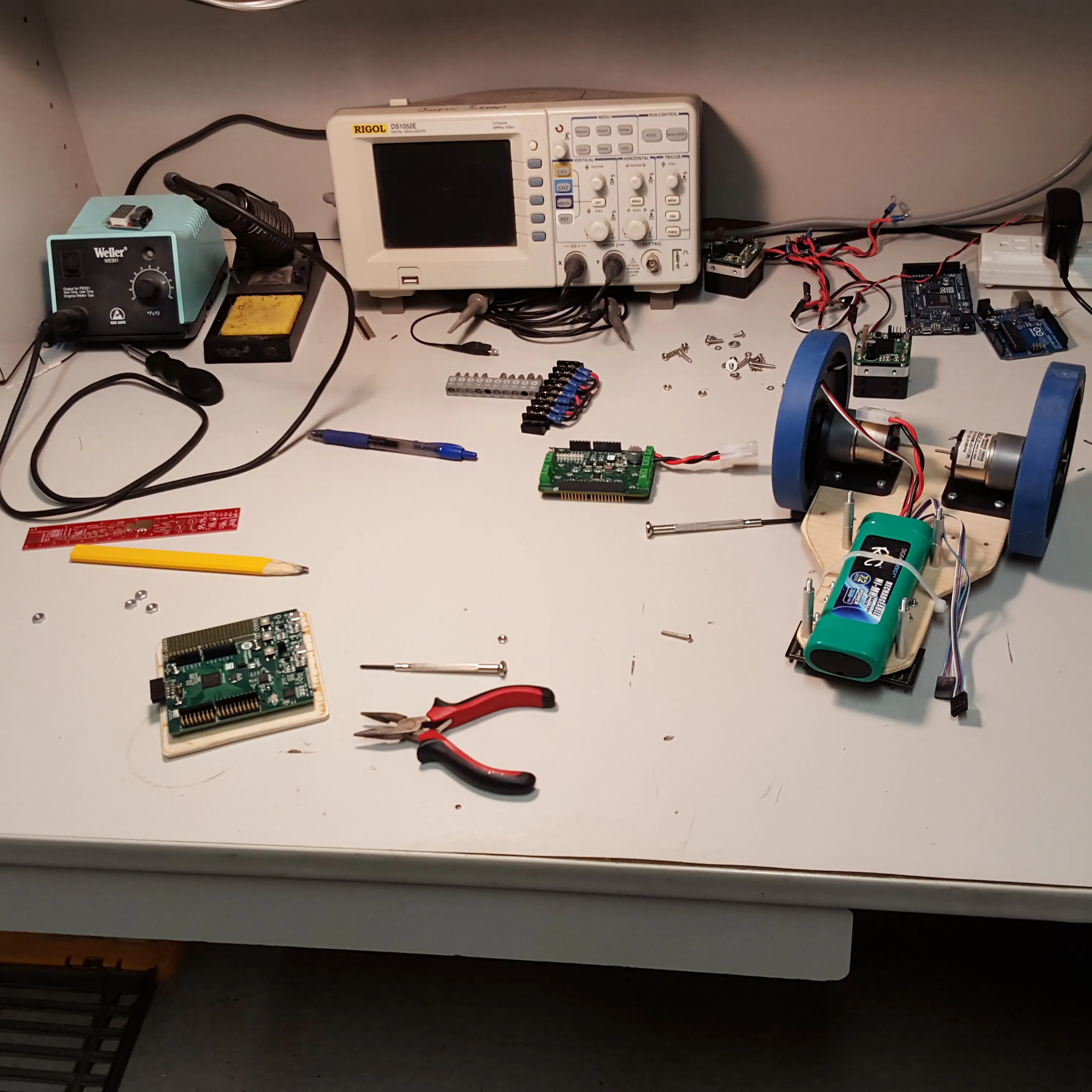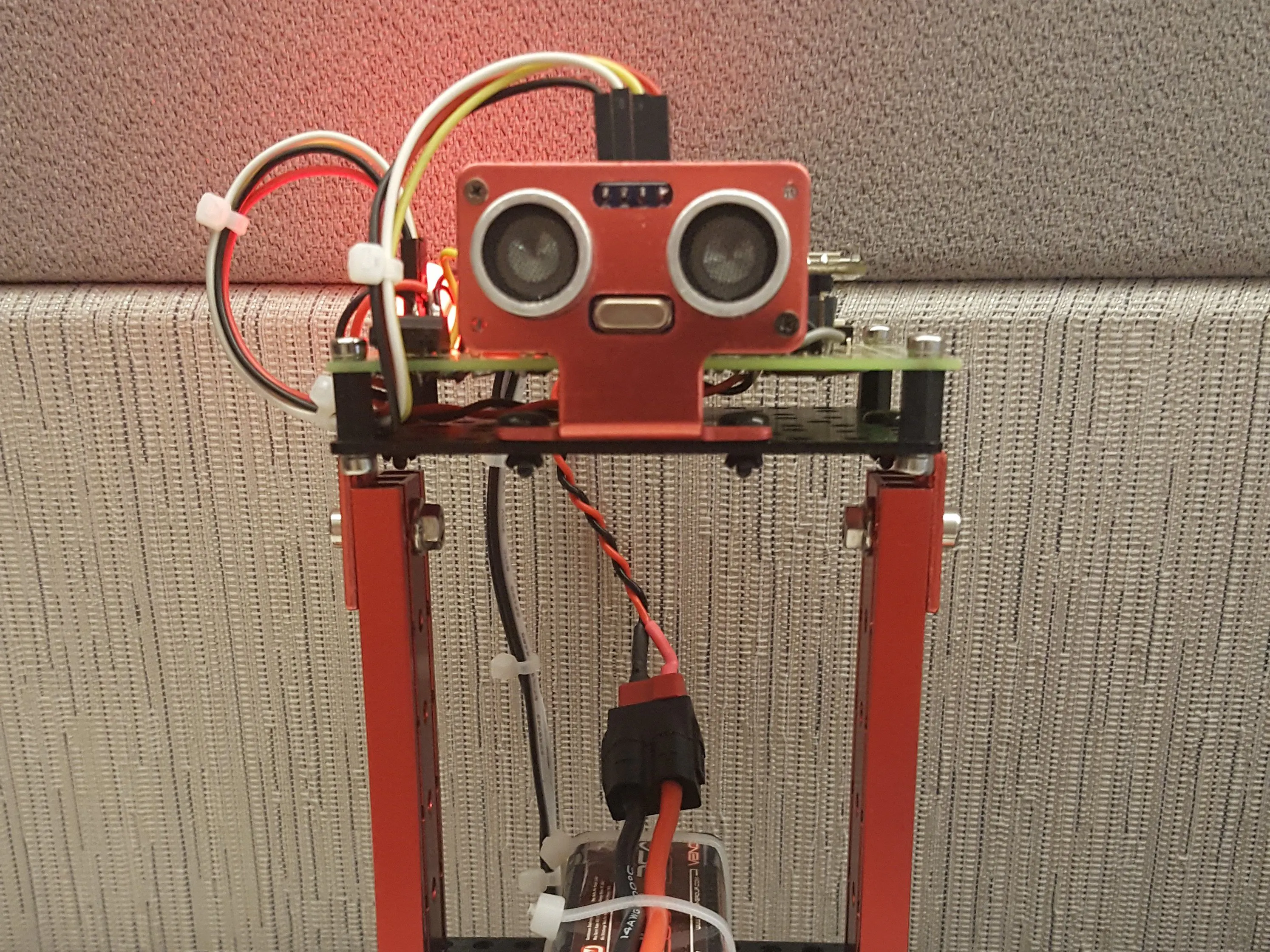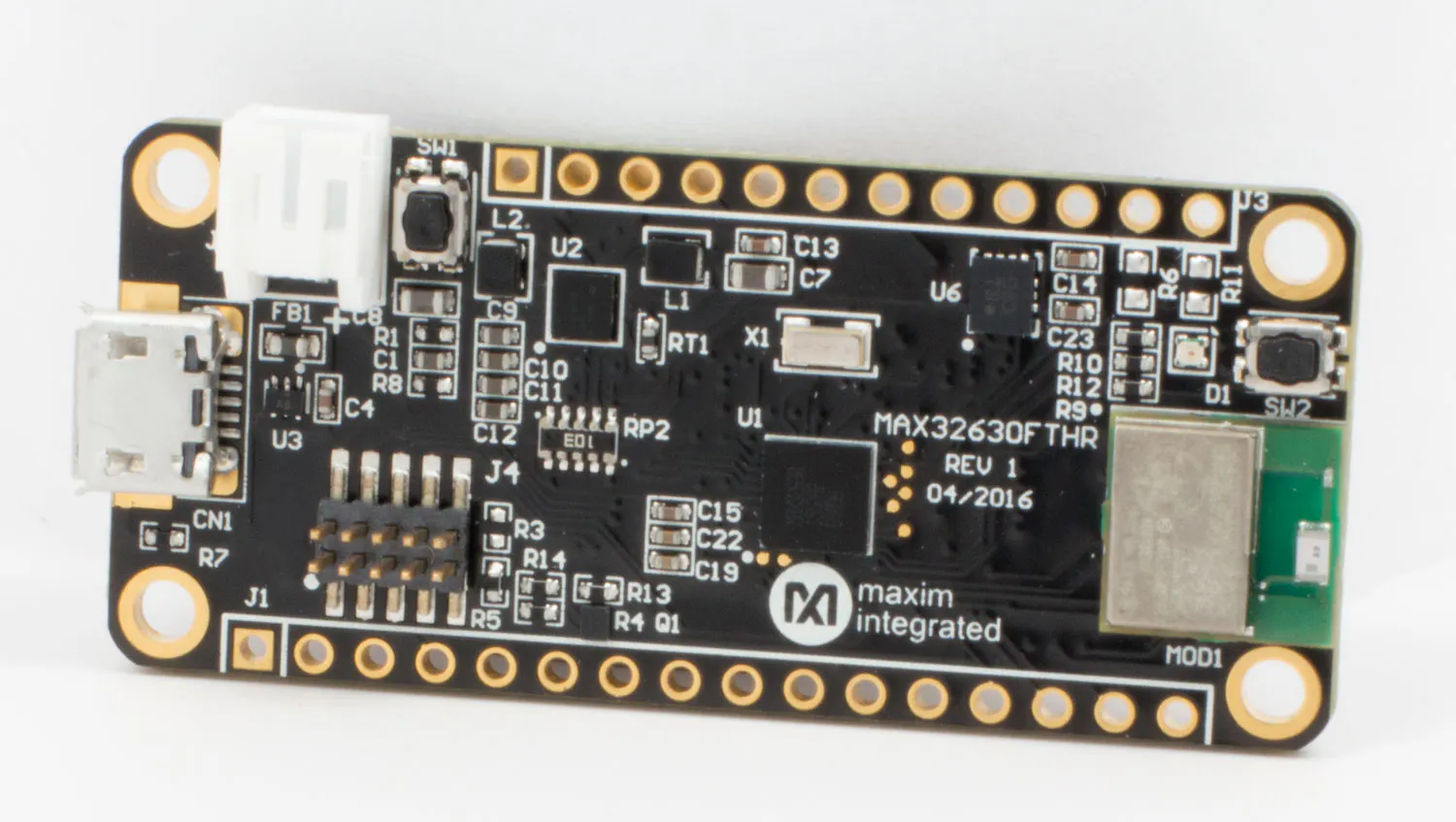# MAX32630FTHR Balance Bot

Balancing robot using the MAX32630FTHR which has an on-board Bosch Sensortec BMI160 IMU.

IntermediateWork in progress1,744## Things used in this project

### Hardware componentsMaxim Integrated MAX32630FTHR
×1
 Pololu - MAX14870 Single Brushed DC Motor Driver Carrier
×2
 OSEPP - 2-Wheeler Balancing Robot Kit
×1
 Venom 2000mAh 2S LiPo Battery Pack
×1

## Code

### MAX32630FTHR Balance Bot

C/C++
MBED program for MAX32630FTHR implementing the control loop and data logging.
```/**********************************************************************
*
* Permission is hereby granted, free of charge, to any person obtaining a
* copy of this software and associated documentation files (the "Software"),
* to deal in the Software without restriction, including without limitation
* the rights to use, copy, modify, merge, publish, distribute, sublicense,
* and/or sell copies of the Software, and to permit persons to whom the
* Software is furnished to do so, subject to the following conditions:
*
* The above copyright notice and this permission notice shall be included
* in all copies or substantial portions of the Software.
*
* THE SOFTWARE IS PROVIDED "AS IS", WITHOUT WARRANTY OF ANY KIND, EXPRESS
* OR IMPLIED, INCLUDING BUT NOT LIMITED TO THE WARRANTIES OF
* MERCHANTABILITY, FITNESS FOR A PARTICULAR PURPOSE AND NONINFRINGEMENT.
* IN NO EVENT SHALL MAXIM INTEGRATED BE LIABLE FOR ANY CLAIM, DAMAGES
* OR OTHER LIABILITY, WHETHER IN AN ACTION OF CONTRACT, TORT OR OTHERWISE,
* ARISING FROM, OUT OF OR IN CONNECTION WITH THE SOFTWARE OR THE USE OR
* OTHER DEALINGS IN THE SOFTWARE.
*
* Except as contained in this notice, the name of Maxim Integrated
* Products, Inc. shall not be used except as stated in the Maxim Integrated
* Products, Inc. Branding Policy.
*
* The mere transfer of this software does not imply any licenses
* property whatsoever. Maxim Integrated Products, Inc. retains all
* ownership rights.
**********************************************************************/

/*References
*
* Circuit Cellar Issue 316 November 2016
* BalanceBot: A Self-Balancing, Two-Wheeled Robot
*
* Reading a IMU Without Kalman: The Complementary Filter
* http://www.pieter-jan.com/node/11
*
* The Balance Filter
*
*/

#include "mbed.h"
#include "max32630fthr.h"
#include "bmi160.h"
#include "SDFileSystem.h"

float compFilter(float K, float pitch, float gyroX, float accY, float accZ, float DT);

void saveData(uint32_t numSamples, float * loopCoeffs, float * gyroX,
float * accY, float * accZ, int32_t * pw);

//Setup interrupt in from imu
InterruptIn imuIntIn(P3_6);
bool drdy = false;
void imuISR()
{
drdy = true;
}

//Setup start/stop button
DigitalIn startStopBtn(P2_3, PullUp);
InterruptIn startStop(P2_3);
bool start = false;
void startStopISR()
{
start = !start;
}

//Setup callibrate button
DigitalIn callibrateBtn(P5_2, PullUp);
InterruptIn callibrate(P5_2);
bool cal = false;
void callibrateISR()
{
cal = !cal;
}

//Ping sensor trigger
DigitalOut pingTrigger(P3_2, 0);
Ticker pingTriggerTimer;

void triggerPing()
{
pingTrigger = !pingTrigger;
wait_us(1);
pingTrigger = !pingTrigger;
}

DigitalIn p51(P5_1, PullNone);
InterruptIn pingEchoRiseInt(P5_1);
DigitalIn p45(P4_5, PullNone);
InterruptIn pingEchoFallInt(P4_5);
Timer pingTimer;

float cm = 0;
const float US_PER_CM = 29.387;

void echoStartISR()
{
pingTimer.reset();
}

void echoStopISR()
{

cm = (us/ US_PER_CM);
}

int main()
{
MAX32630FTHR pegasus;
pegasus.init(MAX32630FTHR::VIO_3V3);

static const float MAX_PULSEWIDTH_US = 40.0F;
static const float MIN_PULSEWIDTH_US = -40.0F;

static const bool FORWARD = 0;
static const bool REVERSE = 1;

//Setup left motor(from driver seat)
DigitalOut leftDir(P5_6, FORWARD);
PwmOut leftPwm(P4_0);
leftPwm.period_us(40);
leftPwm.pulsewidth_us(0);

//Make sure P4_2 and P4_3 are Hi-Z
DigitalIn p42_hiZ(P4_2, PullNone);
DigitalIn p43_hiZ(P4_3, PullNone);

//Setup right motor(from driver seat)
DigitalOut rightDir(P5_4, FORWARD);
PwmOut rightPwm(P5_5);
rightPwm.period_us(40);
rightPwm.pulsewidth_us(0);

//Turn off RGB LEDs
DigitalOut rLED(LED1, LED_OFF);
DigitalOut gLED(LED2, LED_OFF);
DigitalOut bLED(LED3, LED_OFF);

uint32_t failures = 0;

DigitalIn uSDdetect(P2_2, PullUp);
static const uint32_t N = 14400;
uint32_t samples = 0;
float accYaxisBuff[N];
float accZaxisBuff[N];
float gyroXaxisBuff[N];
int32_t pulseWidthBuff[N];

//Setup I2C bus for IMU
I2C i2cBus(P5_7, P6_0);
i2cBus.frequency(400000);

//Get IMU instance and configure it

//Power up sensors in normal mode
if(imu.setSensorPowerMode(BMI160::GYRO, BMI160::NORMAL) != BMI160::RTN_NO_ERROR)
{
printf("Failed to set gyroscope power mode\n");
failures++;
}

wait(0.1);

if(imu.setSensorPowerMode(BMI160::ACC, BMI160::NORMAL) != BMI160::RTN_NO_ERROR)
{
printf("Failed to set accelerometer power mode\n");
failures++;
}
wait(0.1);

BMI160::AccConfig accConfig;
accConfig.range = BMI160::SENS_4G;
accConfig.us = BMI160::ACC_US_OFF;
accConfig.bwp = BMI160::ACC_BWP_2;
accConfig.odr = BMI160::ACC_ODR_11;
if(imu.setSensorConfig(accConfig) == BMI160::RTN_NO_ERROR)
{
{
{
printf("ACC read data desn't equal set data\n\n");
printf("ACC Set Range = %d\n", accConfig.range);
printf("ACC Set UnderSampling = %d\n", accConfig.us);
printf("ACC Set BandWidthParam = %d\n", accConfig.bwp);
printf("ACC Set OutputDataRate = %d\n\n", accConfig.odr);
failures++;
}

}
else
{
printf("Failed to read back accelerometer configuration\n");
failures++;
}
}
else
{
printf("Failed to set accelerometer configuration\n");
failures++;
}

BMI160::GyroConfig gyroConfig;
gyroConfig.range = BMI160::DPS_2000;
gyroConfig.bwp = BMI160::GYRO_BWP_2;
gyroConfig.odr = BMI160::GYRO_ODR_11;
if(imu.setSensorConfig(gyroConfig) == BMI160::RTN_NO_ERROR)
{
{
{
printf("GYRO read data desn't equal set data\n\n");
printf("GYRO Set Range = %d\n", gyroConfig.range);
printf("GYRO Set BandWidthParam = %d\n", gyroConfig.bwp);
printf("GYRO Set OutputDataRate = %d\n\n", gyroConfig.odr);
failures++;
}

}
else
{
printf("Failed to read back gyroscope configuration\n");
failures++;
}
}
else
{
printf("Failed to set gyroscope configuration\n");
failures++;
}

if(failures == 0)
{
printf("ACC Range = %d\n", accConfig.range);
printf("ACC UnderSampling = %d\n", accConfig.us);
printf("ACC BandWidthParam = %d\n", accConfig.bwp);
printf("ACC OutputDataRate = %d\n\n", accConfig.odr);
printf("GYRO Range = %d\n", gyroConfig.range);
printf("GYRO BandWidthParam = %d\n", gyroConfig.bwp);
printf("GYRO OutputDataRate = %d\n\n", gyroConfig.odr);
wait(1.0);

//Sensor data vars
BMI160::SensorData accData;
BMI160::SensorData gyroData;
BMI160::SensorTime sensorTime;

//Complementary Filter vars, filter weight K
static const float K = 0.9978F;
float pitch = 0.0F;

//PID coefficients

//tunning with Wilson, Kc = 4.2, pc = 0.166

static const float DT = 0.00125F;
static const float KP = 2.5F;
static const float KI = (30.0F*DT);
static const float KD = (0.05F/DT);
float setPoint = 0.0F;
float loopCoeffs[] = {setPoint, K, KP, KI, KD, DT};

//Control loop vars
float currentError, previousError;
float integral = 0.0F;
float derivative = 0.0F;
float pulseWidth;

//Enable data ready interrupt from imu for constant loop timming
imu.writeRegister(BMI160::INT_EN_1, 0x10);
//Active High PushPull output on INT1
imu.writeRegister(BMI160::INT_OUT_CTRL, 0x0A);
//Map data ready interrupt to INT1
imu.writeRegister(BMI160::INT_MAP_1, 0x80);

//Tie INT1 to callback fx
imuIntIn.rise(&imuISR);

//Tie SW2 to callback fx
startStop.fall(&startStopISR);

//Tie P5_2 to callback fx
callibrate.fall(&callibrateISR);
bool firstCal = true;

//Callbacks for echo measurement
pingEchoRiseInt.fall(&echoStartISR);
pingEchoFallInt.rise(&echoStopISR);

//Timer for echo measurements
pingTimer.start();

//Timer for trigger
pingTriggerTimer.attach(&triggerPing, 0.05);

//Position control vars/constants
//static const float PING_SP = 20.0F;
//static const float PING_KP = 0.0F;
//float pingCurrentError = 0.0F;

//control loop timming indicator
DigitalOut loopPulse(P5_3, 0);

//Count for averaging setPoint offset, 2 seconds of data
uint32_t offsetCount = 0;

while(1)
{

if(cal || (firstCal == true))
{
cal = false;
firstCal = false;
pitch = 0.0F;
setPoint = 0.0F;

rLED = LED_ON;
gLED = LED_ON;

while(offsetCount < 1600)
{
if(drdy)
{
drdy = false;

imu.getGyroAccXYZandSensorTime(accData, gyroData, sensorTime, accConfig.range, gyroConfig.range);

//Feedback Block, pitch estimate in degrees
pitch = compFilter(K, pitch, gyroData.xAxis.scaled, accData.yAxis.scaled, accData.zAxis.scaled, DT);

//Accumalate pitch measurements
setPoint += pitch;
offsetCount++;
}
}

//Average measurements
setPoint = setPoint/offsetCount;
printf("setPoint = %5.2f\n\n", setPoint);
//Clear count for next time
offsetCount = 0;
}

while(start && (pitch > -20.0F) && (pitch < 20.0F))
{
rLED = LED_OFF;
gLED = LED_ON;

if(drdy)
{
//Start, following takes ~456us on MAX32630FTHR with 400KHz I2C bus
//At 1600Hz ODR, ~73% of loop time is active doing something
loopPulse = !loopPulse;

drdy = false;

imu.getGyroAccXYZandSensorTime(accData, gyroData, sensorTime, accConfig.range, gyroConfig.range);

//Feedback Block, pitch estimate in degrees
pitch = compFilter(K, pitch, gyroData.xAxis.scaled, accData.yAxis.scaled, accData.zAxis.scaled, DT);

//PID Block
//Error signal
currentError = (setPoint - pitch);

//Integral term, dt is included in KI
integral = (integral + currentError);

//Derivative term, dt is included in KD
derivative = (currentError - previousError);

//Set right/left pulsewidth
//Just balance for now, so both left/right are the same
pulseWidth = ((KP * currentError) + (KI * integral) + (KD * derivative));

/*
{
//Get error signal
pingCurrentError = PING_SP - cm;
}

pulseWidth += (pingCurrentError * PING_KP);
*/

//Clamp to maximum duty cycle and check for forward/reverse
//based on sign of error signal (negation of pitch since setPoint = 0)
if(pulseWidth > MAX_PULSEWIDTH_US)
{
rightDir = FORWARD;
leftDir = FORWARD;
pulseWidth = 40.0F;
rightPwm.pulsewidth_us(40);
leftPwm.pulsewidth_us(40);
}
else if(pulseWidth < MIN_PULSEWIDTH_US)
{
rightDir = REVERSE;
leftDir = REVERSE;
pulseWidth = -40.0F;
rightPwm.pulsewidth_us(40);
leftPwm.pulsewidth_us(40);
}
else
{
if(pulseWidth < 0.0F)
{
rightDir = REVERSE;
leftDir = REVERSE;
rightPwm.pulsewidth_us(static_cast<uint32_t>(1 - pulseWidth));
leftPwm.pulsewidth_us(static_cast<uint32_t>(1 - pulseWidth));
}
else
{
rightDir = FORWARD;
leftDir = FORWARD;
rightPwm.pulsewidth_us(static_cast<uint32_t>(pulseWidth));
leftPwm.pulsewidth_us(static_cast<uint32_t>(pulseWidth));
}
}

if(samples < N)
{
accYaxisBuff[samples] = accData.yAxis.scaled;
accZaxisBuff[samples] = accData.zAxis.scaled;
gyroXaxisBuff[samples] = gyroData.xAxis.scaled;
pulseWidthBuff[samples] = static_cast<int32_t>(pulseWidth);
samples++;
}

//save current error for next loop
previousError = currentError;

//Stop
loopPulse = !loopPulse;
}
}

if((pitch > 20.0F) || (pitch < -20.0F))
{
start = false;
}

pitch = 0.0F;
integral = 0.0F;
previousError = 0.0F;
rightPwm.pulsewidth_us(0);
leftPwm.pulsewidth_us(0);

rLED = LED_ON;
gLED = LED_ON;
wait(0.25);
rLED = LED_OFF;
gLED = LED_OFF;
wait(0.25);

if(!uSDdetect && (samples > 0))
{
loopCoeffs = setPoint;
bLED = LED_ON;
saveData(samples, loopCoeffs, gyroXaxisBuff, accYaxisBuff, accZaxisBuff, pulseWidthBuff);
samples = 0;
bLED = LED_OFF;
}
}
}
else
{
while(1)
{
rLED = !rLED;
wait(0.25);
}
}
}

//*****************************************************************************
void saveData(uint32_t numSamples, float * loopCoeffs, float * gyroX,
float * accY, float * accZ, int32_t * pw)
{
SDFileSystem sd(P0_5, P0_6, P0_4, P0_7, "sd");  // mosi, miso, sclk, cs
FILE *fp;

fp = fopen("/sd/balBot.txt", "a");
if(fp != NULL)
{
fprintf(fp, "Samples,%d,,,,,\n", numSamples);
fprintf(fp, "Setpoint,%5.2f,,,,,\n", loopCoeffs);
fprintf(fp, "K, %f,,,,,\n", loopCoeffs);
fprintf(fp, "KP, %f,,,,,\n", loopCoeffs);
fprintf(fp, "KI, %f,,,,,\n", loopCoeffs);
fprintf(fp, "KD, %f,,,,,\n", loopCoeffs);
fprintf(fp, "DT, %f,,,,,\n", loopCoeffs);
fprintf(fp, "Time, Y-Acc, Gyro dps, Gyro Estimate, Acc Estimate, Filter Estimate, PW\n");

float accPitch = 0.0F;
float gyroDegrees = 0.0F;
float pitch = 0.0F;
float K = loopCoeffs;
float DT = loopCoeffs;

for(uint32_t idx = 0; idx < numSamples; idx++)
{
fprintf(fp, "%f,", idx*DT);
fprintf(fp, "%5.4f,", accY[idx]);
fprintf(fp, "%6.2f,", gyroX[idx]);
gyroDegrees += (gyroX[idx] * DT);
fprintf(fp, "%5.2f,", gyroDegrees);
accPitch = ((180.0F/3.14159F) * atan(accY[idx]/accZ[idx]));
fprintf(fp, "%5.2f,", accPitch);
pitch = compFilter(K, pitch, gyroX[idx], accY[idx], accZ[idx], DT);
fprintf(fp, "%5.2f,", pitch);
fprintf(fp, "%d", pw[idx]);
fprintf(fp, "\n");
}
fprintf(fp, "\n");
fclose(fp);
}
else
{
printf("Failed to open file\n");
}
}

//*****************************************************************************
float compFilter(float K, float pitch, float gyroX, float accY, float accZ, float DT)
{
return ((K * (pitch + (gyroX * DT))) + ((1.0F - K) * ((180.0F / 3.1459F) * atan(accY/accZ))));
}
```

### SciLab Script

MATLAB
Says MATLAB for language, however, is a SciLab script used to process data recorded to SD card on robot.
```//Plotting fx for MAX32630FTHR Balance Bot
//Load into scilab console with following command
//exec('balBotPlot.sce', -1);
//Use by giving path to botData.txt
//plotData('path/to/txt/file.txt');

function plotData(dataPath)

//open file and get first seven lines that contain coeffs
f = mopen(dataPath, 'r');
//get first 7 lines of text data
textData = mgetl(f, 8);
mclose(f);

//Parse this data, print, and assign to var
//Probably a better way to do this, but this worked
for idx = 1:7

line = strsplit(textData(idx), ',');
loopConst = strtod(line(2));

select idx
case 1 then
mprintf('Samples = %d\n', loopConst);
samples = loopConst;
case 2 then
mprintf('Setpoint = %5.2f\n', loopConst);
SP = loopConst;
case 3 then
mprintf('K = %5.4f\n', loopConst);
K = loopConst;
case 4 then
mprintf('KP = %5.4f\n', loopConst);
KP = loopConst;
case 5 then
mprintf('KI = %6.4f\n', loopConst);
KI = loopConst;
case 6 then
mprintf('KD = %5.4f\n', loopConst);
KD = loopConst;
case 7 then
mprintf('DT = %7.6f\n', loopConst);
DT = loopConst;
else
break
end
end

setPointVector = samples+1:1;
for idx = 1:samples+1
setPointVector(idx) = SP;
end

zeroVector = zeros((samples + 1), 1);

timeVector = data(8:(samples + 8), 1);
accYaxisVector = data(8:(samples + 8), 2);
gyroDPSVector = data(8:(samples + 8), 3);
gyroEstVector = data(8:(samples + 8), 4);
accEstVector = data(8:(samples + 8), 5);
filterEstVector = data(8:(samples + 8), 6);
pwVector = data(8:(samples + 8), 7);

fig1 = scf();
fig1.figure_name = "Accelerometer_Data.pdf";
subplot(2,1,1);
plot(timeVector, accYaxisVector);
xtitle("y-acc vs Time", "Time(sec)", "y-acc(g)");

subplot(2,1,2);
plot(timeVector, accEstVector);
xtitle("ATAN(y-acc/z-acc) vs Time", "Time(sec)", "Angle(deg)");

fig2 = scf();
fig2.figure_name = "Gyroscope_Data.pdf";
subplot(2,1,1);
plot(timeVector, gyroDPSVector);
xtitle("x-gyro vs Time", "Time(sec)", "Gyro(deg/sec)");

subplot(2,1,2);
plot(timeVector, gyroEstVector);
xtitle("Numeric Integration x-gyro vs Time", "Time(sec)", "Angle(deg)");

fig3 = scf();
fig3.figure_name = "Gyro/Filter_Estimates.pdf";
plot(timeVector, [gyroEstVector, filterEstVector, setPointVector]);
xtitle("Gyro and Filter Angle Estimates", "Time(sec)", "Angle(deg)");
legend("Gyro Estimate", "Filtered Estimate", "SetPoint", [-5]);

fig4 = scf();
fig4.figure_name = "y-Acc_x-Gyro_Angle_Estimates.pdf";
subplot(3,1,1);
plot(timeVector, accYaxisVector);
xtitle("y-acc vs Time", "Time(sec)", "y-acc(g)");

subplot(3,1,2);
plot(timeVector, gyroDPSVector);
xtitle("x-gyro vs Time", "Time(sec)", "Gyro(deg/sec)");

subplot(3,1,3);
plot(timeVector, [gyroEstVector, accEstVector, filterEstVector]);
xtitle("Accel, Gyro, Filter Estimates", "Time(sec)", "Angle(deg)");
legend("Gyro Estimate", "Acc Estimate", "Filtered Estimate", [-5]);

fig5 = scf();
fig5.figure_name = "Angle_Estimates.pdf";
plot(timeVector, [gyroEstVector, accEstVector, filterEstVector, setPointVector]);
xtitle("Accel, Gyro, Filter Estimates", "Time(sec)", "Angle(deg)");
legend("Gyro Estimate", "Acc Estimate", "Filtered Estimate", "SetPoint", [-5]);

fig6 = scf();
fig6.figure_name = "PulseWidth_Filter_Output.pdf";
subplot(2,1,1);
plot(timeVector, [pwVector, zeroVector]);
xtitle("PulseWidth vs Time", "Time(sec)", "PulseWidth(us)");

subplot(2,1,2);
plot(timeVector, [filterEstVector, setPointVector]);
xtitle("Feedback vs Time", "Time(sec)", "Angle(deg)");

endfunction
```

## Credits

### Justin Jordan

2 projects • 2 followers
Industrial technician turned engineer.